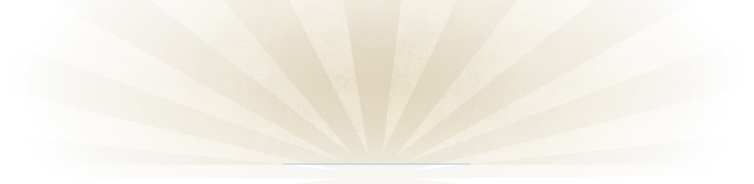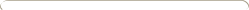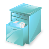﻿ Friden (Singer) EC 1117Details:

This electronic calculator contains early Hitachi MOS LSI integrated circuits including a digital memory and a display of nixie tubes.

There is a mechanical construction to visualize the places of the decimal point and the thousands.  The electronic decimal point can be determined with the bottom rotary switch.  If during calculation an overflow arises this decimal point starts floating.

Usage:

Enter a number, then pushing  “ += “ adds the number to the result register.  “- =“ subtracts in the same way.

The “M1+” and “M1-”  key work similarly in memory.

Multiplication:

Enter the 1st number, push the “X” key, enter the 2nd number, push the “+=” or the  “- =“  key.

Division:

Similar to multiplication using the “:” key.

Function of some keys, switches etc.

• The lower rotary key determines the number of digits behind the decimal point. In case of overflow, the decimal point moves to the right.
• The upper rotary key determines special operations:
• 0 :      normal position
• 1:    automatically sums results of multiplications and divisions in memory
• K:      the 2nd number entered will be used as a constant in multiplications and divisions
• 1K: automatically sums in memory while using the 2nd number entered  as a constant
• C” clears the machine except memory
• CE” clears last entry
• T1” brings memory to display & resets memory
• S1brings memory to display without resetting memory
• R”  swaps the current operandsClick to view a full size picture (opens in a new tab)Serial number:

Built in:

Functions:

Power:

Market-introduction:

Production until:

Price:

29447A

Approx. 1971 (20661 seems to be produced early 1971)